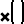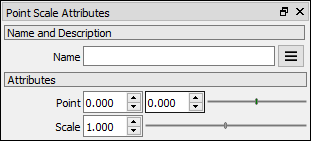# Point Scale node

Left Toolbar >Math Nodes >Point Scale

The Point Scale node allows you to execute a uniform scaling transformation of a point value.

## Procedure

### To execute a point scale operation in the node graph:

1. On the left toolbar choose Point Nodes> Point Scale2. Drag the Point Scale icon into the Node Graph.3. Connect the point Node to the input.

4. Set the scale factor.

5. Connect the Output: result .

## Inputs

point A POINT type of value.

scale A NUMBER type of value.

## Outputs

result

A POINT type of value.(x,y)

## Attributes PanelPoint

This attribute is exposed as input by default. Right-click the Node to hide this attribute as input and set the point values manually.

Scale This attribute is exposed as input by default. Value 1. Right-click the Node to hide this attribute as input and set the value manually.

Math Nodes

Main Page